Attribute Gage Uncertainty
Dr Jody Muelaner posted on May 03, 2019 |
How to determine the accuracy and capability of gages such as go/no-go gauges.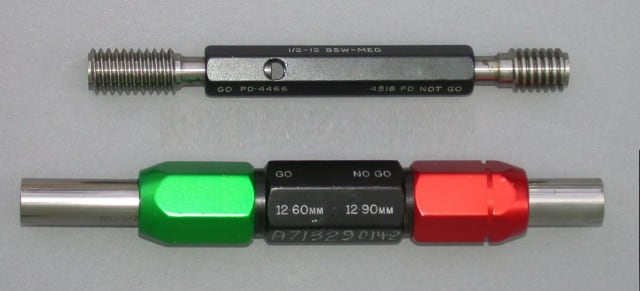I’ve written a lot about how to evaluate the uncertainty measurements. My articles have ranged from basic introductions to metrology and uncertainty budgets, through to more advanced topics such as sensitivity coefficients and Monte Carlo simulation. To date, all of the examples I’ve used have been for variable gages. These are measurement instruments that give a numerical measurement result, on a scale, dial or digital display. Attribute gages are a different type of instrument that gives a binary pass/fail measurement result. Examples of attribute gages include go/no-go plug gages, slip gages and many visual inspection processes.

If you were to apply my previous instructions on uncertainty evaluation directly to an attribute gage, it is likely that you would have some difficulty and possibly even conclude that this is not possible. The difficulty arises in evaluating the repeatability and reproducibility of these measurements. Since they do not produce a numerical result,it is not possible to calculate a standard deviation directly from repeated measurements of a single part. By extension of this, the normal gage R&R ANOVA methods will also not work. However, as you will learn in this article, it is possible to calculate repeatability from a series of repeated measurements.

Attribute gages are very common in manufacturing quality control. It is therefore important to understand the accuracy and capability of these measurements. As I have discussed previously, uncertainty evaluation is the most rigorous way to evaluate the “accuracy” of a measurement.

### Uncertainty Recap

Uncertainty evaluation involves the consideration of all the quantities, or factors, influencing the measurement result. The uncertainty of each individual influence is evaluated. A mathematical model is used to evaluate how these influences combine to produce the final measurement result. There are number of ways to perform these calculations,but the simplest and most intuitive is generally to use an uncertainty budget.

An uncertainty budget is a table that lists each influence quantity on an individual row. There are individual columns for:

• The names of each influence quantity.
• The numerical value of the uncertainty for each influence. For example, a standard deviation, range or half-width.
• The probability distribution which describes each uncertainty.
• Divisors that convert all of the values into standard deviations.
• Sensitivity coefficients that represent the effect the influence has on the final measurement result.
• The standard uncertainty for each influence. This is the uncertainty of the measurement that results from the influence being considered.

Most uncertainty budgets will include some standard influences. These include calibration uncertainty, repeatability and environmental factors. Each sources’ uncertainty may be evaluated using Type-A or Type-B methods. Type-A simply means the statistical evaluation of repeated measurements while Type-B is any other method. Repeatability is almost always evaluated as a Type-A uncertainty. For a simple repeatability study of a variable gage, this simply means measuring the same part a number of times and calculating the standard deviation of the results. Calibration uncertainty is usually evaluated as a Type-B uncertainty by taking the uncertainty value from a calibration certificate.

Uncertainty evaluation for attribute gages follows the same process as for variable gages. The major difference is that the statistical evaluation of repeated measurements to give numerical values for repeatability, and also bias, is considerably more complex.

### Testing the Accuracy of an Attribute gage

The basic principle of a repeatability study for an attribute gage is best understood through an example with a simplified statistical analysis. This can be considered as an accuracy test as it does not go as far as evaluating all sources of uncertainty in the gage. Remember that an attribute gage produces a binary pass/fail measurement result. A given attribute gage, therefore, has a single threshold value—parts with dimensions on one side of this threshold should be passed, and parts with dimensions on the other side of the threshold should be failed. The direction of pass and fail depends on the type of gage.

It is common for gages to be arranged in pairs to measure whether a feature is within a tolerance. For example, when checking the diameter of a hole a go/no-go plug gage may be used. This has a slightly smaller plug at one end, which will “go” into a hole that is larger than the lower specification limit. At the other end it has a larger plug, which will “no-go” (it won’t fit) if the hole is smaller than the upper specification limit. Therefore, if a hole is within tolerance, the go end will go into the hole and the no-go end will not go into the hole. Each end of a go/no-go gage must be considered as a separate gage, requiring its own uncertainty evaluation.

In this example we will consider the calibration of a go plug gage designed to test a nominally 12mm diameter hole with an H8 tolerance. This means that to be in tolerance, the hole diameter must be between 12.000mm and 12.027mm. The go end of a go/no-go gage should, therefore, fit into any hole that is greater than 12.000mm in diameter. This is the threshold value that must be tested.

The first step in the test is to obtain a number of calibrated holes that are close to the threshold value. We might, therefore, inspect a number of machined holes with a high accuracy coordinate measurement machine (CMM) and select eleven holes, ranging in diameter from 11.995mm to 12.005mm with 0.001mm increments. We would then randomly sort the test pieces containing the holes and present them to an inspector for measurement one at a time. The inspector would insert the gage into each hole and state whether the gage goes into the hole or not, i.e. whether the gage would pass or fail the hole. We would record this result next to the calibrated value of the hole. This process is repeated a number of times for each test piece, typically 25 times. Yes, I know, this is a pretty tedious exercise.

The final data obtained, in the form of a table or tally chart, records how many times each size of hole is passed or failed by the gage. If there is negligible uncertainty associated with the repeatability of the gage, then we would expect all of the holes that are smaller than 12mm to fail and all of the holes that are larger than 12mm to pass. We will also get this result if the increments between our test pieces was too large. In such an event, we could either repeat the test we improved test pieces or assume a worst case uncertainty.

If we have chosen sensible increments for the calibrated test pieces, and there is a significant repeatability uncertainty, then we will see a result something like the below: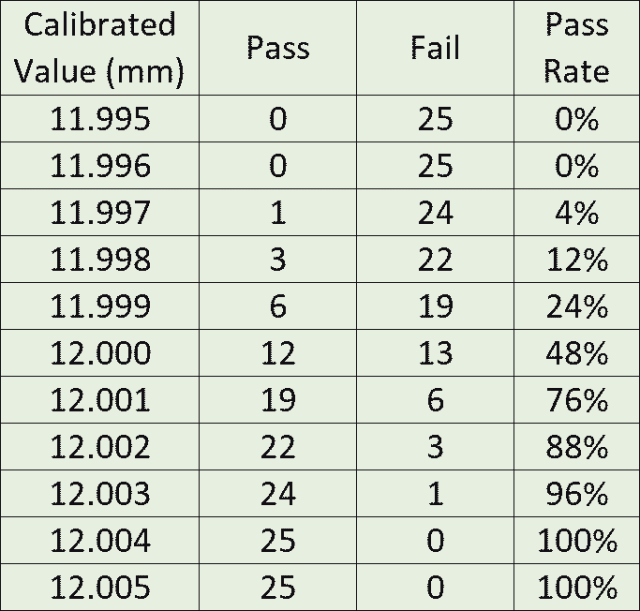Results of accuracy test.

It is clear from these results that when the diameter of the hole is very close to the transitional value, the gage does not give a repeatable result. The simplest statistical analysis of these results would be to take the range of values between zero percent pass rate and 100 percent pass rate. In this case, this would give an accuracy of +/- 0.004mm.

### Determining Standard Deviation for an Attribute gage

Looking at the test results given above, it isn’t obvious that a standard deviation can be calculated from this data. It is, however, possible to estimate the standard deviation by fitting a probability distribution to the observations. If we plot the results, then an “S” curve can be seen, which closely follows a plot of the cumulative normal probability density function (PDF).Test results plotted together with a fitted cumulative normal distribution

If we can find the mean and standard deviation for the normal distribution that fits to our data, then we can determine the repeatability and bias of the gage. This can be achieved using Excel.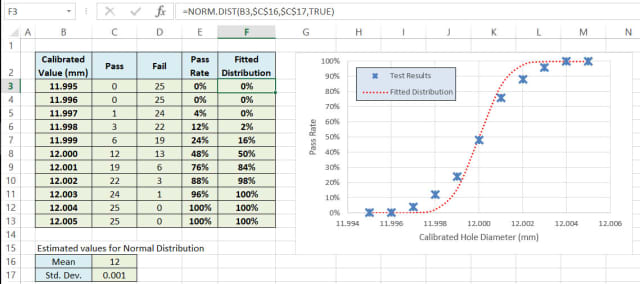Excel Spreadsheet setup to fit a normal distribution (cumulative PDF) to the test results. The chart has the calibrated values from column B on its x-axis. The test results, converted to a percentage pass rate in column E, and the fitted distribution in column F, are both plotted against these calibrated values. The fitted distribution references the calibrated values in column B as well as estimates for the mean (C16) and standard deviation (C17).

In the above example, the fitted distribution uses the Excel function NORM.DIST with the cumulative PDF option (the final argument is set to true). The formulas in column F references the corresponding value for the calibrated value in column B. The formulas in column F also reference the estimated values for the mean (C16) and standard deviation (C17). Note the use of the dollar signs for the estimated values. This allows the formulas to be dragged down the rows and always reference the same values.

Note that the fitted distribution in the above example is not a very close fit to the test results. This is because initial estimates have been used for the mean and standard deviation. One way to improve the fit would be to manually change these values and observe how the fit can be improved. If you want to get a feel for this, you could duplicate the above spreadsheet and give it a go. It’s quite difficult to.

A more efficient way to fit the distribution to the test data is to minimize the sum of the squared differences, or residuals. To do this, add another column and enter a simple formula to calculate the difference between the fitted distribution and the test results. In this example, cell G3 contains the formula =F3-E3,and this formula is then copied down column G. In row 14, the sum of the squares of these residuals is then calculated using the formula =SUMSQ(G3:G13). The distribution can then be fitted by optimizing the values of the mean and standard deviation so that the sum of the squared residuals is minimized. This type of optimization can be carried out automatically using the Solver, found on the DATA tab. Note that the Solver is an add-in which is included with Excel but not turned on by default. If you don’t see the option, you will need to turn it on.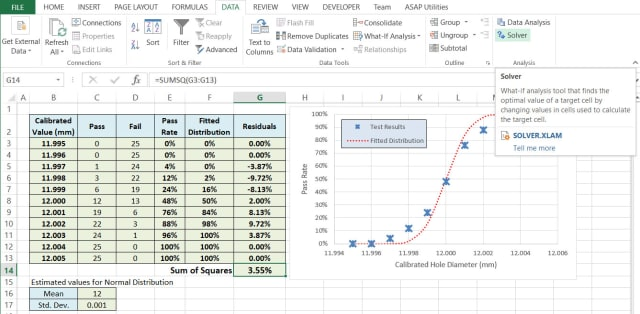Example spreadsheet with column added to calculate residuals and the sum of their squares. The button to launch the solver is also shown.

After selecting the solver button, a dialogue box w ill open to enter the solver parameters. The objective is to minimize the sum of the squared residuals in G14. This is to be performed by changing variable cells in C16:C17 (the mean and standard deviation). Other options can be left as default using a GRG Nonlinear solver and no constraints.Enter the objective and variable cells to change.

When you select Solve, you should see the Sum of Squares value reduce and the fit visibly improved on the chart. A dialogue will open asking whether you want to keep the solver solution, click OK. In the example, the estimated standard deviation has changed significantly. This now appears to be a good estimate for the repeatability of the gage. The difference between the estimated mean and the nominal mean is orders of magnitude smaller than the standard deviation. Therefore it is safe to consider the bias as not being significant without applying any more sophisticated tests for significance. This is an area I will cover in more detail later.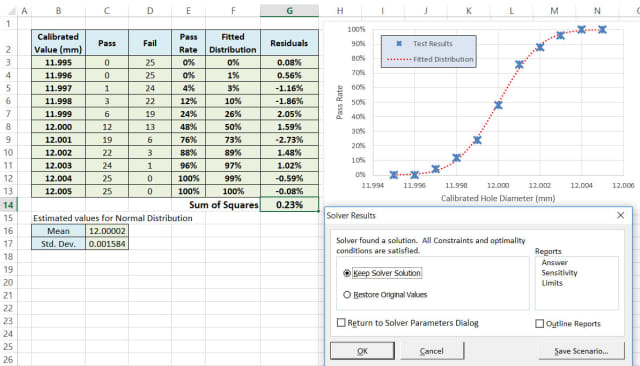Accepting the solver solution.

If you didn’t get a good solution, there are a few possible reasons for this:

1. One common possibility is that the calibrated values do not cover the range of the probability distribution with sufficient resolution. It is usually possible to spot this by looking at the plot of the test results. If this is because the repeatability uncertainty is very small, then it may be acceptable to use a worst-case estimate, as described above, rather than fitting a probability distribution. If this is not appropriate, then additional calibrated samples will need to be obtained and further testing carried out using these.
2. Another common cause of the solver failing is that the initial starting values are not sufficiently close to the optimal solution. You should always start with a manual estimate with a mean roughly in the middle of the test results and a standard deviation that is of the right order of magnitude. Using the chart as a visual sanity check is useful here.
3. Another possibility is that the uncertainty does not follow a normal distribution. If you’re still struggling to get a good fit it, is worth considering this an attempt to fit some other distributions.

I’ll cover some of the finer points of attribute gage studies and uncertainty evaluation in a future post. This more advanced topics include fitting additional probability distributions, refining the resolution of calibrated test samples and testing for the significance of bias detected by the analysis.

 Recommended For You